Note: This is a working paper which will be expanded/updated frequently. All suggestions for improvement are welcome. The directory deleeuwpdx.net/pubfolders/penalty has a pdf version, a html version, the bib files, the complete Rmd file with the code chunks, and the R source code.

# Introduction

Full-dimensional Scaling (FDS) was introduced by De Leeuw (1993). De Leeuw, Groenen, and Mair (2016) discuss it in some detail. In FDS we minimize the usual Multidimensional Scaling (MDS) least squares loss function first used by Kruskal (1964a) and Kruskal (1964b). $\begin{equation}\label{E:stress} \sigma(Z)=\frac12\mathop{\sum\sum}_{1\leq i<j\leq n}w_{ij}(\delta_{ij}-d_{ij}(Z))^2 \end{equation}$ over all $$n\times n$$ configuration matrices $$Z$$. The loss at $$Z$$ is often called the stress of configuration $$Z$$. More generally we define pMDS as the problem of minimizing $$\eqref{E:stress}$$ over all $$n\times p$$ matrices. Thus FDS is the same as nMDS. If a configuration $$Z$$ has $$n$$ columns (i.e. is square) it is called a full configuration.

In $$\eqref{E:stress}$$ the matrices $$W=\{w_{ij}\}$$ and $$\Delta=\{\delta_{ij}\}$$ of weights and dissimilarities are non-negative, symmetric, and hollow. To simplify matters we suppose both $$W$$ and $$\Delta$$ have positive off-diagonal elements. The matrix $$D(X)=\{d_{ij}(Z)\}$$ has the Euclidean distances between the rows of the configuration $$Z$$. Thus $d_{ij}(Z)=\sqrt{(z_i-z_j)'(z_i-z_j)}.$ We now introduce some standard MDS notation, following De Leeuw (1977). Define the matrix $$V=\{v_{ij}\}$$ by $\begin{equation}\label{E:V} v_{ij}=\begin{cases}-w_{ij}&\text{ if }i\not= j,\\\sum_{j=1}^n w_{ij}&\text{ if }i=j,\end{cases} \end{equation}$ and the matrix valued function $$B(Z)=\{b_{ij}(Z)\}$$ by $\begin{equation}\label{E:B} b_{ij}(Z)=\begin{cases}-w_{ij}e_{ij}(Z)&\text{ if }i\not= j,\\\sum_{j=1}^n w_{ij}e_{ij}(Z)&\text{ if }i=j,\end{cases} \end{equation}$ where $$E(Z)=\{e_{ij}(Z)\}$$ is defined as $\begin{equation}\label{E:E} e_{ij}(Z)=\begin{cases}\frac{\delta_{ij}}{d_{ij}(Z)}&\text{ if }d_{ij}(Z)>0,\\0&\text{ otherwise}.\end{cases} \end{equation}$

Note that $$V$$ and $$B(Z)$$ are both positive semi-definite and doubly-centered. Matrix $$V$$ has rank $$n-1$$. If all off-diagonal $$d_{ij}(Z)$$ are positive then $$B(Z)$$ has rank $$n-1$$ for all $$Z$$. Note that De Leeuw (1984) established that near a local minimum of stress all off-diagonal distances are indeed positive. The only vectors in the null-space of both $$V$$ and $$B(Z)$$ are the vectors proportional to the vector with all elements equal to one.

We assume in addition, without loss of generality, that $\frac12\mathop{\sum\sum}_{1\leq i<j\leq n}w_{ij}\delta_{ij}^2=1.$ With these definitions we can rewrite the stress $$\eqref{E:stress}$$ as $\begin{equation}\label{E:mstress} \sigma(Z)=1-\mathbf{tr}\ Z'B(Z)Z+\frac12\mathbf{tr}\ Z'VZ, \end{equation}$ and we can write the stationary equations as $\begin{equation}\label{E:stationary} (V-B(Z))Z=0, \end{equation}$ or, in fixed point form, $$Z=V^+B(Z)Z$$.

Equation $$\eqref{E:mstress}$$ shows, by the way, something which is already obvious from $$\eqref{E:stress}$$. Distances are invariant under translation. This is reflected in $$B(Z)$$ and $$V$$ being doubly-centered. As a consequence we usually require, again without loss of generality, that $$Z$$ is column-centered. And that implies that $$Z$$ has rank at most $$n-1$$, which means that FDS is equivalent to minimizing stress over all $$n\times (n-1)$$ matrices, which we can assume to be column-centered as well. Configurations with $$n-1$$ columns can be called full configurations as well. In addition, distances are invariant under rotation, and consequently if $$Z$$ solves the stationary equations with value $$\sigma(Z)$$ then $$ZK$$ solves the stationary equations for all rotation matrices $$K$$, and $$\sigma(ZK)=\sigma(K)$$. This means there are no isolated local minima in configuration space, each local minimum is actually a continuum of rotated matrices in $$\mathbb{R}^{n\times n}$$. This is a nuisance in the analysis of FDS and pMDS that is best dealt with by switching to the parametrization outlined in De Leeuw (1993).

# Convex FDS

Instead of defining the loss function $$\eqref{E:stress}$$ on the space of all $$n\times n$$ configuration matrices $$Z$$ we can also define it over the space of all positive semidefinite matrices $$C$$ of order $$n$$. This gives $\begin{equation}\label{E:cmds} \sigma(C)=1-\mathop{\sum\sum}_{1\leq i<j\leq n}w_{ij}\delta_{ij}\sqrt{c_{ii}+c_{jj}-2c_{ij}}+\frac12\mathbf{tr}\ VC. \end{equation}$ The Convex Full-dimensional Scaling (CFDS) problem is to minimize loss function $$\eqref{E:cmds}$$ over all $$C\gtrsim 0$$. Obviously if $$Z$$ minimizes $$\eqref{E:stress}$$ then $$C=ZZ'$$ minimizes $$\eqref{E:cmds}$$. And, conversely, if $$C$$ minimizes $$\eqref{E:cmds}$$ then any $$Z$$ such that $$C=ZZ'$$ minimizes $$\eqref{E:stress}$$.

The definition $$\eqref{E:cmds}$$ shows that the CFDS loss function is a convex function on the cone of positive semi-definite matrices, because the square root of a non-negative linear function of the elements of $$C$$ is concave. Positivity of the weights and dissimilarities implies that loss is actually strictly convex. The necessary and sufficient conditions for $$C$$ to be the unique solution of the CFDS problem are simply the conditions for a proper convex function to attain its minimum at $$C$$ on a closed convex cone (Rockafellar (1970), theorem 31.4). \begin{align*} V-B(C)&\gtrsim 0,\\ C&\gtrsim 0,\\ \mathbf{tr}\ C(V-B(C))&=0. \end{align*} The conditions say that $$C$$ and $$V-B(C)$$ must be positive semi-definite and have complimentary null spaces.

By the same reasoning as in the full configuration case, we also see that CFDS is equivalent to maximizing $$\eqref{E:cmds}$$ over all doubly-centered positive semi-definite matrices.

If $$C$$ is the solution of the CFDS problem then $$\mathbf{rank}(C)$$ is called the Gower rank of the MDS problem defined by $$W$$ and $$\Delta$$ (De Leeuw (2016)). Although there is a unique Gower rank associated with each CFDS problem, we can also talk about the approximate Gower rank by ignoring the small eigenvalues of $$C$$.

# FDS using SMACOF

The usual SMACOF algorithm can be applied to FDS as well. The iterations start with $$Z^{(0)}$$ and use the update rule $\begin{equation}\label{E:smacof} Z^{(k+1)}=V^+B(Z^{(k)})Z^{(k)}, \end{equation}$ where $$V^+$$ is the Moore-Penrose inverse of $$V$$, and is consequently also doubly-centered. This means that all $$Z^{(k)}$$ in the SMACOF sequence, except possibly $$Z^{(0)}$$, are column-centered and of rank at most $$n-1$$. Equation $$\eqref{E:smacof}$$ also shows that if $$Z^{(0)}$$ is of rank $$p<n-1$$ then all $$Z^{(k)}$$ are of rank $$p$$ as well.

De Leeuw (1977) shows global convergence of the SMACOF sequence for pMDS, generated by $$\eqref{E:smacof}$$, to a stationary point, i.e. a point satisfying $$(V-B(Z))Z=0$$. This result also applies, of course, to nMDS, i.e. FDS. If $$Z$$ is a solution of the stationary equations then with $$C=ZZ'$$ we have both $$(V-B(C))C=0$$ and $$C\gtrsim 0$$, but since we generally do not have $$V-B(Z)\gtrsim 0$$, this does not mean that $$C$$ solves the CFDS problem.

In fact, suppose the unique CMDS solution has Gower rank $$r\geq 2$$. Start the SMACOF FDS iterations $$\eqref{E:smacof}$$ with $$Z^{(0)}$$ of the form $$Z^{(0)}=\begin{bmatrix}X^{(0)}&\mid&0\end{bmatrix}$$, where $$X^{(0)}$$ is an $$n\times p$$ matrix of rank $$p<r$$. All $$Z^{(k)}$$ will be of this form and will also be of rank $$p$$, and all accumulation points $$Z$$ of the SMACOF sequence will have this form and $$\mathbf{rank}(Z)\leq p$$. Thus $$C=ZZ'$$ cannot be the solution of the CMDS problem.

The next result shows that things are allright, after all. Although stress in FDS is certainly not a convex function of $$Z$$, it remains true that all local minima are global.

Lemma 1: [Expand] If FDS stress has a local minimum at $$\begin{bmatrix}X&\mid&0\end{bmatrix}$$, where $$X$$ is $$n\times p$$ and the zero block is $$n\times q$$ with $$q>1$$, then

1: $$\mathcal{D}\sigma(X)=(V-B(X))X=0$$.

2: $$\mathcal{D}^2\sigma(X)\gtrsim 0$$.

3: $$V-B(X)\gtrsim 0$$.

Proof: We use the fact that stress is differentiable at a local minimum (De Leeuw (1984)). If $$Z=\begin{bmatrix}X&\mid&0\end{bmatrix}+\epsilon\begin{bmatrix}P&\mid&Q\end{bmatrix}$$ then we must have $$\sigma(Z)\geq\sigma(X)$$ for all $$P$$ and $$Q$$. Now $\begin{multline}\label{E:expand} \sigma(Z)=\sigma(X)+\epsilon\ \text{tr}\ P'\mathcal{D}\sigma(X)\ +\\+\frac12\epsilon^2\ \mathcal{D}^2\sigma(X)(P,P)+\frac12\epsilon^2\ \text{tr}\ Q'(V-B(X))Q+o(\epsilon^2). \end{multline}$ The lemma follows from this expansion. $$\blacksquare$$

Theorem 1: [FDS Local Minima] If stationary point $$Z$$ of FDS is a local minimum, then it also is the global minimum, and $$C=ZZ'$$ solves the CFDS problem.

Proof: We start with a special case. Suppose $$Z$$ is a doubly-centered solution of the FDS stationary equations with $$\mathbf{rank}(Z)=n-1$$. Then $$(V-B(Z))Z=0$$ implies $$V=B(Z)$$, which implies $$\delta_{ij}=d_{ij}(Z)$$ for all $$i,j$$. Thus $$\sigma(Z)=0$$, which obviously is the global minimum.

Now suppose $$Z$$ is a doubly-centered local minimum solution of the FDS stationary equations with $$\mathbf{rank}(Z)=r<n-1$$. Without loss of generality we assume $$Z$$ is of the form $$Z=\begin{bmatrix}X&\mid&0\end{bmatrix}$$, with $$X$$ an $$n\times r$$ matrix of rank $$r$$. For $$C=ZZ'$$ to be a solution of the CFDS problem it is necessary and sufficient that $$V-B(Z)\gtrsim 0$$. Lemma 1 shows that this is indeed the case at a local minimum. $$\blacksquare$$

Corrollary 1: [Saddle] A pMDS solution of the stationary equations with $$Z$$ singular is a saddle point.

Corrollary 2: [Nested] Solutions of the stationary equations of pMDS are saddle points of qMDS with $$q>p$$.

The proof of lemma 1 shows that for any $$n\times p$$ configuration $$Z$$, not just for solutions of the FDS stationary equations, if $$V-B(Z)$$ is indefinite we can decrease loss by adding another dimension. If $$Z$$ is a stationary point and $$V-B(Z)$$ is positive semi-definite then we actually have found the CFDS solution, the Gower rank, and the global minimum (De Leeuw (2014)).

# Penalizing Dimensions

In Shepard (1962a) and Shepard (1962b) a nonmetric multidimensional scaling technique is developed which minimizes a loss function over configurations in full dimensionality $$n-1$$. In that sense the technique is similar to FDS. Shepard’s iterative process aims to maintain monotonicity between distances and dissimilarities and at the same time concentrate as much of the variation as possible in a small number of dimensions (De Leeuw (2017)).

Let us explore the idea of concentrating variation in $$p<n-1$$ dimensions, but use an approach which is quite different from the one used by Shepard. We remain in the FDS framework, but we aim for solutions in $$p<n-1$$ dimensions by penalizing $$n-p$$ dimensions of the full configuration, using the classical Courant quadratic penalty function.

Partition a full configuration $$Z=\begin{bmatrix}X&\mid&Y\end{bmatrix}$$, with $$X$$ of dimension $$n\times p$$ and $$Y$$ of dimension $$n\times(n-p)$$. Then $\begin{equation}\label{E:part} \sigma(Z)=1-\mathbf{tr}\ X'B(Z)X - \mathbf{tr}\ Y'B(Z)Y+\frac12 \mathbf{tr}\ X'VX+\frac12 \mathbf{tr}\ Y'VY. \end{equation}$ Also define the penalty term $\begin{equation}\label{E:tau} \tau(Y)=\frac12\mathbf{tr}\ Y'VY, \end{equation}$ and penalized stress $\begin{equation}\label{E:pi} \pi(Z,\lambda)=\sigma(Z)+\lambda\ \tau(Y). \end{equation}$

Our proposed method is to minimize penalized stress over $$Z$$ for a sequence of values $$0=\lambda_1<\lambda_2<\cdots\lambda_m$$. For $$\lambda=0$$ this is simply the FDS problem, for which we know we can compute the global minimum. For fixed $$0<\lambda<+\infty$$ this is a Penalized FDS or PFDS problem. PFDS problems with increasing values of $$\lambda$$ generate a trajectory $$Z(\lambda)$$ in configuration space.

The general theory of exterior penalty functions, which we review in appendix A of this paper, shows that increasing $$\lambda$$ leads to an increasing sequence of stress values $$\sigma$$ and a decreasing sequence of penalty terms $$\tau$$. If $$\lambda\rightarrow+\infty$$ we approximate the global minimum of the FDS problem with $$Z$$ of the form $$Z=\begin{bmatrix}X&\mid&0\end{bmatrix}$$, i.e. of the pMDS problem. This assumes we do actually compute the global minimum for each value of $$\lambda$$, which we hope we can do because we start at the FDS global minimum, and we slowly increase $$\lambda$$. There is also a local version of the exterior penalty result, which implies that $$\lambda\rightarrow\infty$$ takes us to a local minimum of pMDS, so there is always the possibility of taking the wrong trajectory to a local minimum of pMDS.

## Local Minima

The stationary equations of the PFDS problem are solutions to the equations \begin{align} (V-B(Z))X&=0,\\ ((1+\lambda)V-B(Z))Y&=0. \end{align}

We can easily related stationary points and local minima of the FDS and PFDS problem.

Theorem 2: [PFDS Local Minima]

1: If $$X$$ is a stationary point of the pMDS problem then $$Z=[X\mid 0]$$ is a stationary point of the PFDS problem, no matter what $$\lambda$$ is.

2: If $$Z=[X\mid 0]$$ is a local minimum of the PFDS problem then $$X$$ is a local minimum of pMDS and $$(1+\lambda)V-B(X)\gtrsim 0$$, or $$\lambda\geq\|V^+B(X)\|_\infty-1$$, with $$\|\bullet\|_\infty$$ the spectral radius (largest eigenvalue).

Proof:

Part 1 follows by simple substitution in the stationary equations.

Part 2 follows from the expansion for $$Z=[X+\epsilon P\mid\epsilon Q]$$. $\begin{multline}\label{E:expand2} \pi(Z)=\pi(X)+\epsilon\ \text{tr}\ P'\mathcal{D}\sigma(X)\ +\\+\frac12\epsilon^2\ \mathcal{D}^2\sigma(X)(P,P)+\frac12\epsilon^2\ \text{tr}\ Q'((1+\lambda)V-B(X))Q+o(\epsilon^2). \end{multline}$ At a local minimum we must have $$\mathcal{D}\sigma(X)=0$$ and $$\mathcal{D}^2\sigma(X)(P,P)\gtrsim 0$$, which are the necessary conditions for a local minimum of pMDS. We also must have $$((1+\lambda)V-B(X))\gtrsim 0$$. $$\blacksquare$$

Note that the conditions in part 2 of theorem 2 are also sufficient for PFDS to have a local minimum at $$[X\mid 0]$$, provided we eliminate translational and rotational indeterminacy by a suitable reparametrization, as in De Leeuw (1993).

# Algorithm

The SMACOF algorithm for penalized stress is a small modification of the unpenalized FDS algorithm $$\eqref{E:smacof}$$. We start our iterations for $$\lambda_j$$ with the solution for $$\lambda_{j-1}$$ (the starting solution for $$\lambda_1=0$$ can be completely arbitrary). The update rules for fixed $$\lambda$$ are

\begin{align} X^{(k+1)}&=V^+B(Z^{(k)})X^{(k)},\\ Y^{(k+1)}&=\frac{1}{1+\lambda}V^+B(Z^{(k)})Y^{(k)}. \end{align}

Thus we compute the FDS update $$Z^{(k+1)}=V^+B(Z^{(k)})Z^{(k)}$$ and then divide the last $$n-p$$ columns by $$1+\lambda$$.

Code is in the appendix. Let us analyze a number of examples.

# Examples

This section has a number of two-dimensional and a number of one-dimensional examples. The one-dimensional examples are of interest, because of the documented large number of local minima of stress in the one-dimensional case, and the fact that for small and medium $$n$$ exact solutions are available (for example, De Leeuw (2005)). By default we use seq(0, 1, length = 101) for $$\lambda$$ in most examples, but for some of them we dig a bit deeper and use longer sequences with smaller increments.

If for some value of $$\lambda$$ the penalty term drops below the small cutoff $$\gamma$$, for example 10^{-10}, then there is not need to try larger values of $$\lambda$$, because they will just repeat the same result. We hope that result is the global minimum of the 2MDS problem.

The output for each example is a table in which we give, the minimum value of stress, the value of the penalty term at the minimum, the value of $$\lambda$$, and the number of iterations needed for convergence. Typically we print for the first three, the last three, and some regularly spaced intermediate values of $$\lambda$$. Remember that the stress values increase with increasing $$\lambda$$, and the penalty values decrease.

For two-dimensional examples we plot all two-dimensional configurations, after rotating to optimum match (using the function matchMe() from the appendix). We connect corresponding points for different values of $$\lambda$$. Points corresponding to the highest value of $$\lambda$$ are labeled and have a different plot symbol. For one-dimensional examples we put 1:n on the horizontal axes and plot the single dimension on the vertical axis, again connecting corresponding points. We label the points corresponding with the highest value of $$\lambda$$, and draw horizontal lines through them to more clearly show their order on the dimension.

The appendix also has code for the function checkUni(), which we have used to check the solutions in the one dimensional case are indeed local minima. The function checks the necessary condition for a local minimum $$x=V^+u$$, with $u_i=\sum_{j=1}^nw_{ij}\delta_{ij}\ \mathbf{sign}\ (x_i-x_j).$ It should be emphasized that all examples are just meant to study convergence of penalized FDS. There is no interpretation of the MDS results

## Chi Squares

In this example, of order 10, the $$\delta_{ij}$$ are independent draws from a chi-square distribution with two degrees of freedom. There is no structure in this example, everything is random.

## itel  198 lambda   0.000000 stress 0.175144 penalty 0.321138
## itel    5 lambda   0.010000 stress 0.175156 penalty 0.027580
## itel    3 lambda   0.020000 stress 0.175187 penalty 0.025895
## itel    1 lambda   0.100000 stress 0.175914 penalty 0.015172
## itel    1 lambda   0.200000 stress 0.177666 penalty 0.004941
## itel    4 lambda   0.300000 stress 0.178912 penalty 0.000088
## itel    6 lambda   0.310000 stress 0.178933 penalty 0.000020
## itel   20 lambda   0.320000 stress 0.178939 penalty 0.000000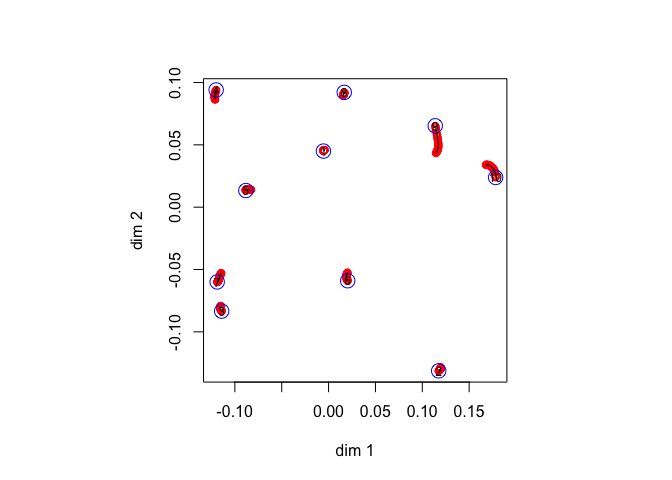It seems that in this example the first two dimensions of FDS are already close to optimal for 2MDS. This is because the Gower rank of the dissimilarities is only three (or maybe four, the fourth singular value of the FDS solution $$Z$$ is very small).

## Regular Simplex

The regular simplex has all dissimilarities equal to one. We use an example with $$n=10$$, for which the global minimum (as far as we know) of pMDS with $$p=2$$ is a configuration with nine points equally spaced on a circle and one point in the center.

## itel    1 lambda   0.000000 stress 0.000000 penalty 0.400000
## itel    7 lambda   0.010000 stress 0.000102 penalty 0.375457
## itel    5 lambda   0.020000 stress 0.000425 penalty 0.360310
## itel    1 lambda   0.100000 stress 0.008861 penalty 0.258186
## itel    1 lambda   0.200000 stress 0.032285 penalty 0.149502
## itel    1 lambda   0.300000 stress 0.060136 penalty 0.076344
## itel    1 lambda   0.400000 stress 0.082466 penalty 0.035994
## itel    1 lambda   0.500000 stress 0.098777 penalty 0.013130
## itel    1 lambda   0.600000 stress 0.107410 penalty 0.002747
## itel    1 lambda   0.700000 stress 0.109651 penalty 0.000258
## itel    1 lambda   0.790000 stress 0.109872 penalty 0.000013
## itel    1 lambda   0.800000 stress 0.109876 penalty 0.000009
## itel   65 lambda   0.810000 stress 0.109880 penalty 0.000000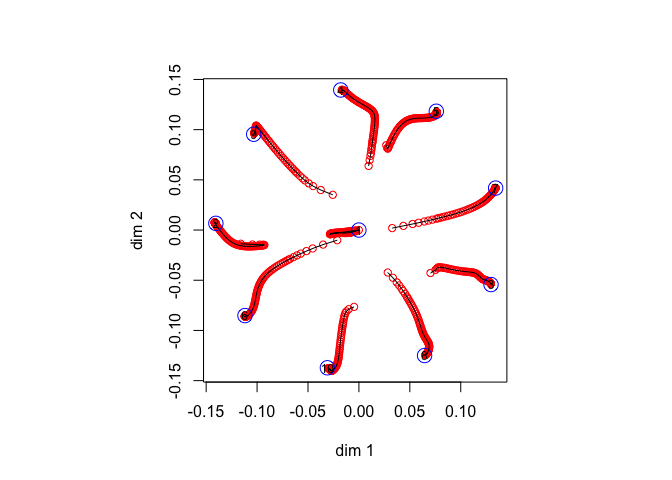Next, we look at the regular simplex with $$n=4$$, for which the global minimum has four points equally spaced on a circle (i.e. in the corners of a square). We use seq(0, 1, length = 101) for the $$\lambda$$ sequence.

## itel    1 lambda   0.000000 stress 0.000000 penalty 0.250000
## itel    2 lambda   0.010000 stress 0.000035 penalty 0.162295
## itel    1 lambda   0.020000 stress 0.000122 penalty 0.158591
## itel    1 lambda   0.100000 stress 0.003808 penalty 0.122344
## itel    1 lambda   0.200000 stress 0.014089 penalty 0.084111
## itel    1 lambda   0.300000 stress 0.028044 penalty 0.053366
## itel    1 lambda   0.400000 stress 0.043331 penalty 0.028965
## itel    1 lambda   0.500000 stress 0.056851 penalty 0.011482
## itel    1 lambda   0.600000 stress 0.064718 penalty 0.002471
## itel    1 lambda   0.700000 stress 0.066799 penalty 0.000203
## itel    1 lambda   0.800000 stress 0.066982 penalty 0.000005
## itel    1 lambda   0.820000 stress 0.066985 penalty 0.000002
## itel    1 lambda   0.830000 stress 0.066986 penalty 0.000001
## itel    1 lambda   0.840000 stress 0.066986 penalty 0.000001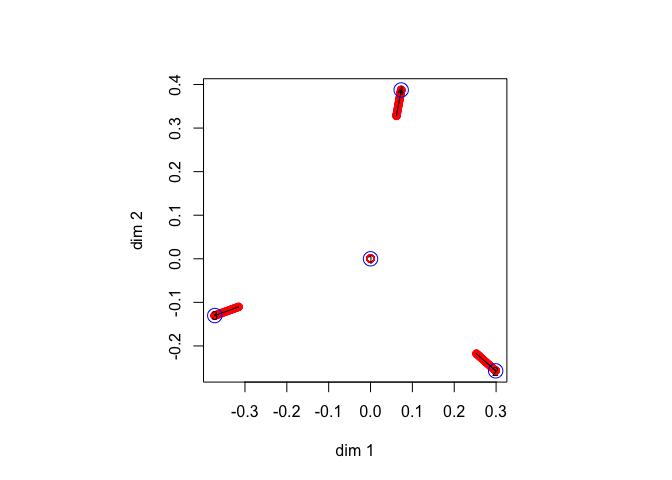The solution converges to an equilateral triangle with the fourth point in the centroid. This is a local minimum. What basically happens is that the first two dimensions of the FDS solution are too close to the local minimum. Or, what amounts to the same thing, the Gower rank is too large (it is $$n-1$$ for a regular simplex) , there is too much variation in the higher dimensions, and as a consequence the first two dimensions of FDS are a bad 2MDS solution. We try to repair this by refining the trajectory, using seq(0, 1, 10001).

## itel    1 lambda   0.000000 stress 0.000000 penalty 0.250000
## itel    2 lambda   0.000100 stress 0.000000 penalty 0.166622
## itel    1 lambda   0.000200 stress 0.000000 penalty 0.166583
## itel    1 lambda   0.202200 stress 0.028595 penalty 0.000001
## itel    1 lambda   0.202300 stress 0.028595 penalty 0.000001
## itel    1 lambda   0.202400 stress 0.028595 penalty 0.000001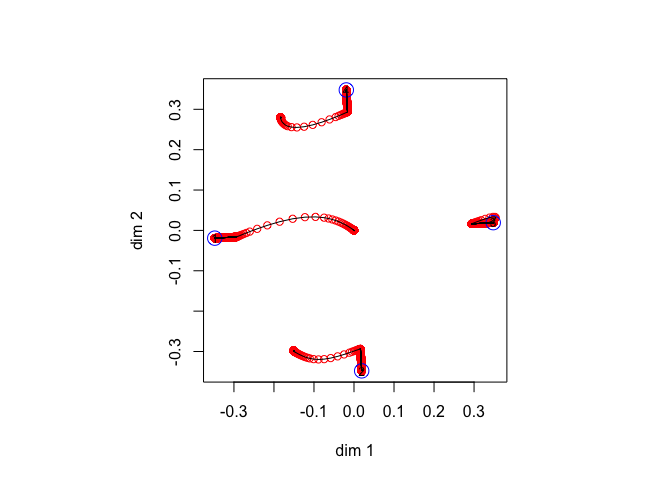Now the trajectories move us from what starts out similar to an equilateral triangle to the corners of the square, and thus we do find the global minimum in this way. It is remarkable that we manage to find the square even when we start closer to the triangle with midpoint.

## Intelligence

These are correlations between eight intelligence tests, taken from the smacof package. We convert to dissimilarities by taking the negative logarithm of the correlations. As in the chi-square example, the FDS and the 2MDS solution are very similar and the PMDS trajectories are short.

## itel 2951 lambda   0.000000 stress 0.107184 penalty 7.988384
## itel    7 lambda   0.010000 stress 0.107560 penalty 0.685654
## itel    4 lambda   0.020000 stress 0.108528 penalty 0.628538
## itel    3 lambda   0.030000 stress 0.110045 penalty 0.573208
## itel    3 lambda   0.040000 stress 0.112449 penalty 0.510730
## itel    2 lambda   0.050000 stress 0.114714 penalty 0.464650
## itel    2 lambda   0.060000 stress 0.117623 penalty 0.415037
## itel    2 lambda   0.070000 stress 0.121095 penalty 0.364536
## itel    2 lambda   0.080000 stress 0.125010 penalty 0.315023
## itel    2 lambda   0.090000 stress 0.129226 penalty 0.267831
## itel    2 lambda   0.100000 stress 0.133589 penalty 0.223898
## itel    2 lambda   0.110000 stress 0.137944 penalty 0.183868
## itel    3 lambda   0.120000 stress 0.143921 penalty 0.133739
## itel    2 lambda   0.130000 stress 0.147473 penalty 0.106166
## itel    4 lambda   0.140000 stress 0.153215 penalty 0.064499
## itel    4 lambda   0.150000 stress 0.157159 penalty 0.037735
## itel    9 lambda   0.160000 stress 0.161434 penalty 0.010337
## itel   72 lambda   0.170000 stress 0.163122 penalty 0.000000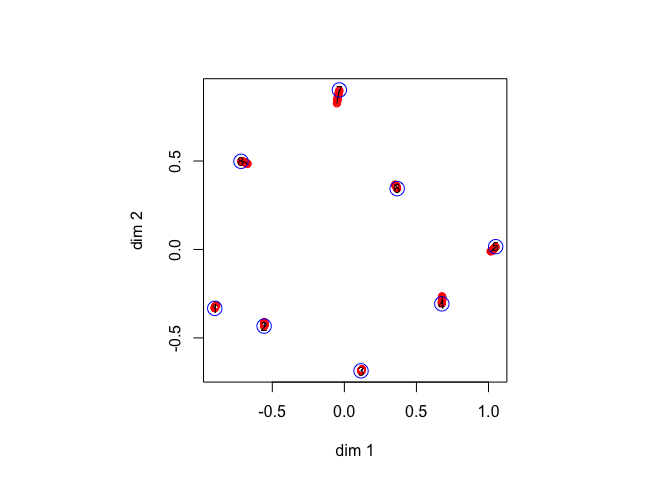The singular values of the FDS solution are 1.78e+00, 1.36e+00, 5.94e-01, 1.47e-01, 3.29e-03, 1.70e-16, 6.62e-17, 4.67e-17, which shows that the Gower rank is probably five, but approximately two.

## Countries

This is the wish dataset from the ’smacof package, with similarities between 12 countries. They are converted to dissimilarties by subtracting each of them from seven.

## itel 1381 lambda   0.000000 stress 4.290534 penalty 98.617909
## itel    4 lambda   0.010000 stress 4.301341 penalty 36.137074
## itel    3 lambda   0.020000 stress 4.336243 penalty 34.389851
## itel    1 lambda   0.100000 stress 5.187917 penalty 23.300775
## itel    1 lambda   0.200000 stress 7.539228 penalty 11.543635
## itel    1 lambda   0.300000 stress 9.901995 penalty 4.963372
## itel    1 lambda   0.400000 stress 11.523357 penalty 1.859569
## itel    1 lambda   0.500000 stress 12.391692 penalty 0.556411
## itel    1 lambda   0.590000 stress 12.696493 penalty 0.080144
## itel    1 lambda   0.600000 stress 12.708627 penalty 0.060113
## itel  100 lambda   0.610000 stress 12.738355 penalty 0.000000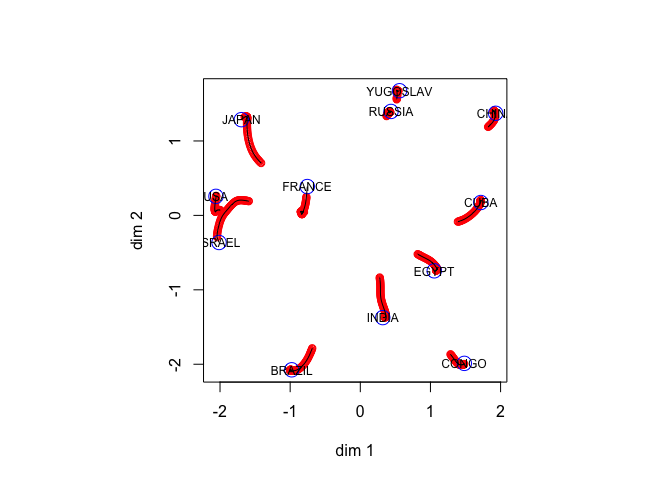The singular values of the FDS solution are 4.20e+00, 3.71e+00, 2.67e+00, 1.80e+00, 1.33e+00, 6.64e-01, 5.97e-04, 4.88e-16, 2.49e-16, 2.10e-16, 1.52e-16, 3.04e-17, and the Gower rank is six or seven.

## Dutch Political Parties

In 1967 one hundred psychology students at Leiden University judged the similarity of nine Dutch political parties, using the complete method of triads (De Gruijter (1967)). Data were aggregated and converted to dissimilarities. We first print the matrix of dissimilarities.

##      KVP   PvdA  VVD   ARP   CHU   CPN   PSP   BP    D66
## KVP  0.000 0.209 0.196 0.171 0.179 0.281 0.250 0.267 0.230
## PvdA 0.209 0.000 0.250 0.210 0.231 0.190 0.171 0.269 0.204
## VVD  0.196 0.250 0.000 0.203 0.185 0.302 0.281 0.257 0.174
## ARP  0.171 0.210 0.203 0.000 0.119 0.292 0.250 0.271 0.228
## CHU  0.179 0.231 0.185 0.119 0.000 0.290 0.263 0.259 0.225
## CPN  0.281 0.190 0.302 0.292 0.290 0.000 0.152 0.236 0.276
## PSP  0.250 0.171 0.281 0.250 0.263 0.152 0.000 0.256 0.237
## BP   0.267 0.269 0.257 0.271 0.259 0.236 0.256 0.000 0.274
## D66  0.230 0.204 0.174 0.228 0.225 0.276 0.237 0.274 0.000

The trajectories from FDS to 2MDS show some clear movement, especially of the D’66 party, which was new at the time.

## itel  223 lambda   0.000000 stress 0.000000 penalty 0.414526
## itel    5 lambda   0.010000 stress 0.000061 penalty 0.196788
## itel    2 lambda   0.020000 stress 0.000199 penalty 0.190472
## itel    1 lambda   0.100000 stress 0.004399 penalty 0.136576
## itel    1 lambda   0.200000 stress 0.015811 penalty 0.075466
## itel    1 lambda   0.300000 stress 0.028235 penalty 0.036636
## itel    1 lambda   0.400000 stress 0.038275 penalty 0.012608
## itel    1 lambda   0.500000 stress 0.043644 penalty 0.002156
## itel    1 lambda   0.520000 stress 0.044091 penalty 0.001324
## itel    1 lambda   0.530000 stress 0.044253 penalty 0.001019
## itel  277 lambda   0.540000 stress 0.044603 penalty 0.000000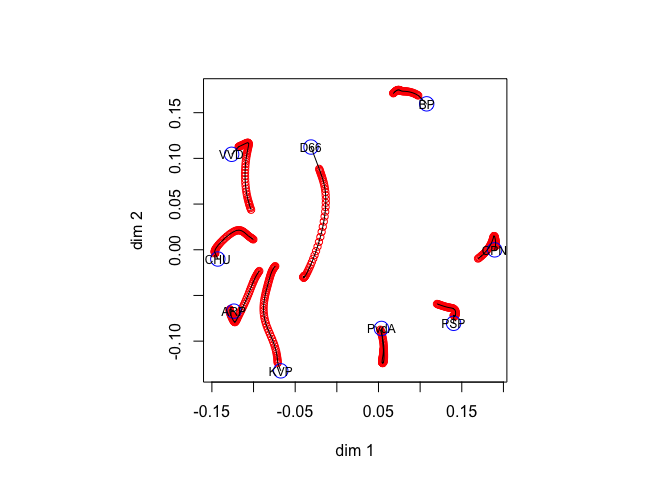There seems to be some bifurcation going on at the end, so we repeat the analysis using seq(0, 1, length = 1001) for $$\lambda$$. The results turn out to be basically the same.

## itel  223 lambda   0.000000 stress 0.000000 penalty 0.414526
## itel    4 lambda   0.001000 stress 0.000001 penalty 0.204225
## itel    2 lambda   0.002000 stress 0.000002 penalty 0.203535
## itel    1 lambda   0.468000 stress 0.044604 penalty 0.000001
## itel    1 lambda   0.469000 stress 0.044604 penalty 0.000000
## itel  166 lambda   0.470000 stress 0.044603 penalty 0.000000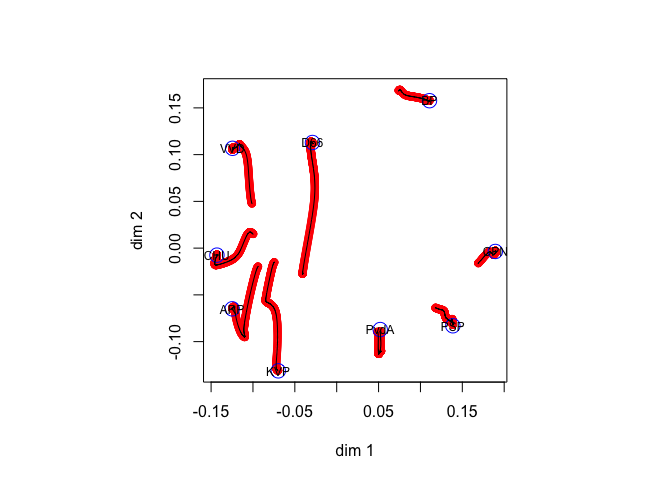The singular values of the FDS solution are 2.95e-01, 2.10e-01, 1.89e-01, 1.34e-01, 1.16e-01, 1.06e-01, 8.61e-02, 7.06e-02, 2.30e-18, and the Gower rank is probably eight. This is mainly because these data, being averages, regress to the mean and thus have a substantial additive constant. If we repeat the analysis after subtracting .1 from all dissimilarities we get basically the same solution, but with somewhat smoother trajectories.

## itel  511 lambda   0.000000 stress 0.000176 penalty 0.150789
## itel    2 lambda   0.001000 stress 0.000176 penalty 0.037759
## itel    2 lambda   0.002000 stress 0.000176 penalty 0.037619
## itel    1 lambda   0.370000 stress 0.007642 penalty 0.000000
## itel    1 lambda   0.371000 stress 0.007642 penalty 0.000000
## itel    1 lambda   0.372000 stress 0.007642 penalty 0.000000`